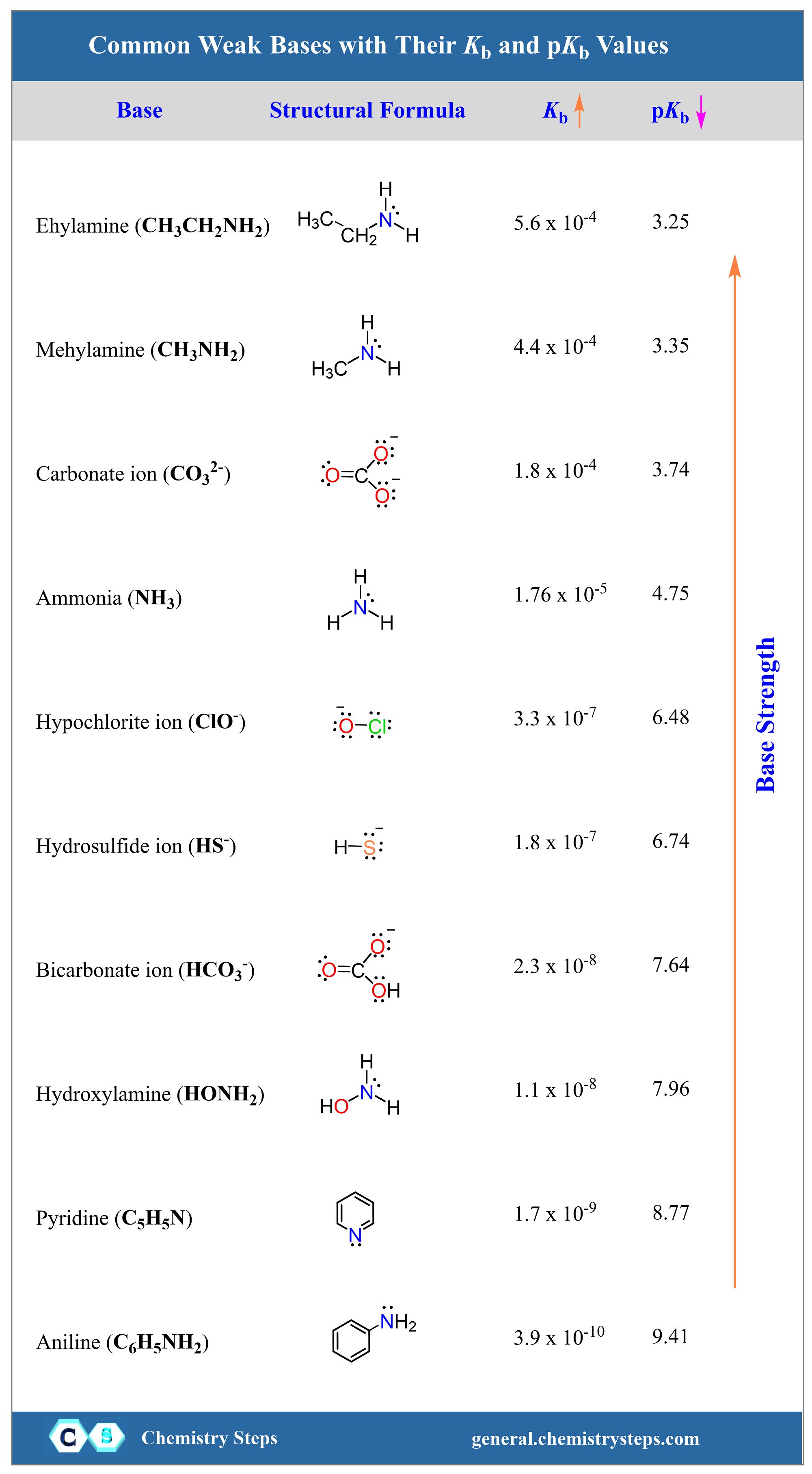# Strong Bases

The most widely used strong bases in general chemistry are the hydroxides of alkali (group 1A) metals such as KOH (caustic or just potash), NaOH (caustic soda), and LiOH.

Just like the strong acids, we recognize them by their ability to completely ionize in aqueous solutions.

For example, if a bottle reads 2.0 M NaOH, it actually indicates that the concentration of hydroxide and sodium ions is 2.0 M each. There is virtually no undissociated NaOH left in the solution as it is almost entirely ionized to ions.

NaOH(aq) → Na+(aq) + OH(aq)

The hydroxides of alkaline earth (group 2A) metals are also considered strong bases, however, not all of them are very soluble in water. Among these, Ca(OH)2, called slaked lime, is the most soluble and least expensive one and is used in making mortars and cement. It is called slaked lime because it is made by treating lime (CaO) with water.

CaO(s) + H2O(l) → Ca(OH)2(aq)

Because of their relatively higher solubility, calculating the concentration of, and therefore, the pH of their solutions, Ca(OH)2, Ba(OH)2, and Sr(OH)2 follow the same principles as the hydroxides of alkali metals. Thus, the solution of 0.25 M Ca(OH)2 will contain 0.25 M Ca2+, and 0.50 M OHions because each mole of Ca(OH)2 ionizes to one mole of Ca2+ and 2 moles of OHions:

Ca(OH)2(aq) → Ca2+(aq) + 2OH(aq)

# Oxides as Bases

All alkali metal and alkaline earth metal oxides, except BeO which is amphoteric, are basic as well because their reaction with water produces the corresponding hydroxide.

Na2O(s) → H2O + 2NaOH(aq)

BaO(s) → H2O + Ba(OH)2(aq)

These as well, are types of acid-base reactions where the base is the oxide ion (O2-) and water is the acid. So, in an ionic form, the reactions can be shown as:

O2-(aq) + H2O(l) → 2OH(aq)

Now, because acid-base reactions always occur in the direction of forming a weaker acid and a base, the oxide ion (O2-) must be a stronger base than the hydroxide ion. Therefore, alkali and alkaline earth metal oxides are stronger bases than the corresponding hydroxides.

# Weak Bases

Unlike strong bases, weak bases do not contain a hydroxide ion. Instead, they produce it by reacting with water. As a general reaction, this can be shown as:

B(aq) + H2O(l ) ⇆ BH+(aq) + OH(aq)

where, B is the weak base, and is its conjugate acid BH+

Notice that the reaction is shown with a double arrow as it proceeds to a little extent until an equilibrium is established.

The equilibrium is characterized by the base-dissociation constant:

${K_{\rm{b}}}\;{\rm{ = }}\;\frac{{\left[ {{\rm{B}}{{\rm{H}}^{\rm{ + }}}} \right]\left[ {{\rm{O}}{{\rm{H}}^{\rm{ – }}}} \right]}}{{\left[ {\rm{B}} \right]}}$

For example, ammonia is a weak base because it produces a hydroxide ion and its conjugate base ammonium ion:

NH3(aq) + H2O(l ) ⇆ NH4+(aq) + OH(aq)

${K_{\rm{b}}}\;{\rm{ = }}\;\frac{{\left[ {{\rm{N}}{{\rm{H}}_{\rm{4}}}^{\rm{ + }}} \right]\left[ {{\rm{O}}{{\rm{H}}^{\rm{ – }}}} \right]}}{{\left[ {{\rm{N}}{{\rm{H}}_{\rm{3}}}} \right]}}$

The most common weak bases are amines, which are the derivatives of ammonia. Some of the examples are methyl amine (CH3NH2), ethyl amine (CH3NH2), hydroxyl amine (HONH2) aniline (C6H5NH2), and pyridine (C5H5N).

Aside from these, the carbonates (CO32-) and bicarbonates (HCO3) are also considered weak bases.

# Kb and pKb as Characteristics of Base Strength

Like any equilibrium reaction, the larger the equilibrium constant, the more the reaction is shifted to the right. The Kb values of the most common weak bases are listed in the table below:Notice that all Kb values are very small which makes it inconvenient for certain calculations or quickly tell which base is stronger or weaker. To simplify the numbers, the negative logarithm of Kb is often used to get rid of the exponent. This is what we also saw when introducing the pH to quantify the acidity of the solution.

pKb = – log Kb

For example, the pKb of ammonia and pyridine are:

pKb (NH3= – log Kb = – log 1.8 x 10-5  = 4.75

pKb (C5H5N= – log Kb = – log 1.7 x 10-9 = 8.77

Based on the Kb values, NH3 is the strongest base, and it has a smaller pKb value. So, just like the acids, the trait is that a stronger base has a lower pKb while the Kb increases with the acid strength.

The larger the Kb, the stronger the base.

The smaller the pKb, the stronger the base.

The pKb values of most common acids are given next to the corresponding Ka values in the table we have shown above.

If you need more details on strong and weak bases in organic chemistry, particularly how amines are used there, check out this post.

Check Also